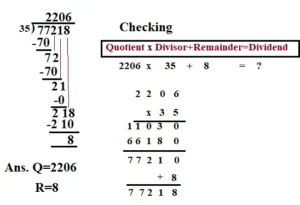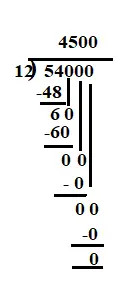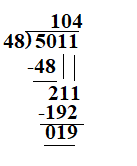October 1, 2023

# Division sums for class 5

Division sums for class 5

Division is one of the most basic arithmetic operations. Students learn division in primary school by dividing a group into small equal portions. Students will learn to compute quotients, remainders, and division word problems by working through these division worksheets.  By practicing these worksheets, students can master the concept of  division.

# Division sums for class 5 with answers pdf

Division Sum for class 5 (Solved examples )

Sol.Example 2.  Mala’s annual income is Rs. 534250 . She spend Rs. 480250 and saves the rest . How much money does she save monthly if she saves an equal amount every month?Sol. Mala’s annual income = Rs. 534250

Amount she spends=  Rs. 480250

Amount she saves = Rs. 534250 -Rs. 480250  = Rs. 54000

Amount she saves every month = Rs. 54000

= Rs. 4500

Example 3.  A book of 1456 pages has 995904 words in it. How many words are there in each page? If each page consists of 38 lines, How many words are there in each line ?

Sol. Number of word in 1456 pages = 995904

number of word in 1 page = 995904 1456

= 684

The number of words in 1 page  =684.

But each page has 38 lines .

The number of words in 38 lines = 684.

The number of words in 1 line =

= 18

Hence, the number of words in each line= 18.

Example 4. The product of two numbers is 1785483. If one of the number is 987 , what is the other number?

Sol. Product of two numbers = 1785483

One number = 987

Other number = 1785483 987

= 1809

Example 5 : 5011 books are to be packed into boxes of 48 books each. How many boxes are completely filled and how many books are left over?

Solution: Total number of books = 5011

number of books in each boxes =48

Number of boxes that are completely filled =  5011Hence 104 boxes are completely filled and 19 books are left.

Example 6: A number was divided by 97; the quotient was 286 and the remainder 14. Find the number.

Sol.  We know that Dividend = (Quotient  x  Divisor ) +remainder

So, the number =  (286 x 97 )+ 14

= 27742+14

=27756

Division Sum for class 5 (Unsolved with answers )

1) There are 98,800 books in a library. How many shelves are there in the library if 400 books can be kept  on 4 selves?

2) Mr. Singh ordered 4680 apple tree saplings. He wants to plant them in 18 rows. How many of them should he plant in each row?

3)  There are 11,050 raw apples. The number of ripe apples is twice the number of raw apples. Mr. Singh packs an equal number of ripe apples in 850 boxes. How many ripe apples will each box contain?

4) The cost of 736 quintals  of wheat is Rs. 1214400 . Find the cost of one quintal of wheat.

5) 187 water tanks of the same capacity can hold 1323025 litres of water. What  is the capacity of each tank ?

6) Toffees are packed in fancy packs of 256 toffees  each. How many packs will be needed to pack 68900 toffees? How many toffees will be left.

7) A number was divided by 97; the quotient was 286 and the remainder 14. Find the number.

8)  44,619 sweets were distributed among 1,000 students of a school. How many sweets will each student get if every student gets an equal number of sweets? How many sweets will be left?

9) A sum of  Rs. 16000 is to be divided equally among 79 persons.  How much will each person  receive and how much will be left over?

10) An army general arranges 7624 soldiers in rows of 36 soldiers  each. How many soldiers are there in each row? How many soldiers are left ?

Ans. 1) 988     2) 260      3)26    4) Rs. 1650   5) 7075 litres  6)  269  ,  36    7)27756   8) 44,  619   9) 202, 42     10) 211, 28

## Division sums for class 5 worksheet

File Name: Division-worksheet-1.pdf

File Name: Division-worksheet-2.pdf

File Name: Division-worksheet-3.pdf

You might also be interested in:#### Bina singh

View all posts by Bina singh →
ssc chsl 2023 tier 1 cut off NIRF Rankings 2023 : Top 10 Engineering colleges in India CBSE Compartment Exam 2023 Application Form SSC CGL 2023 Notification: Important Dates UPSC Recruitment 2023